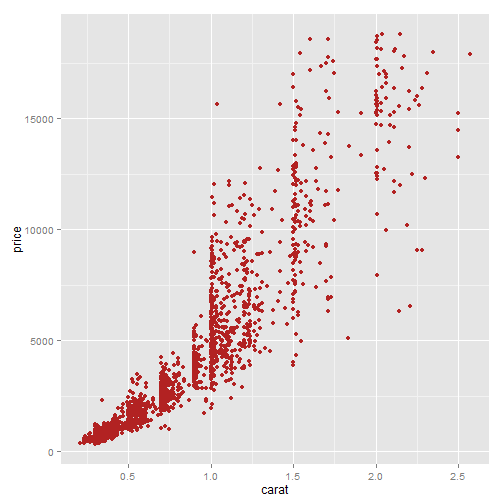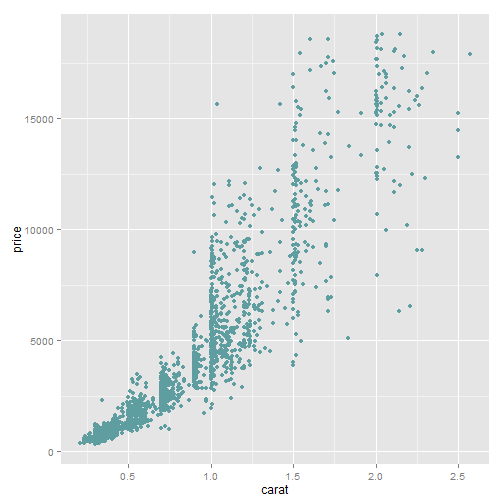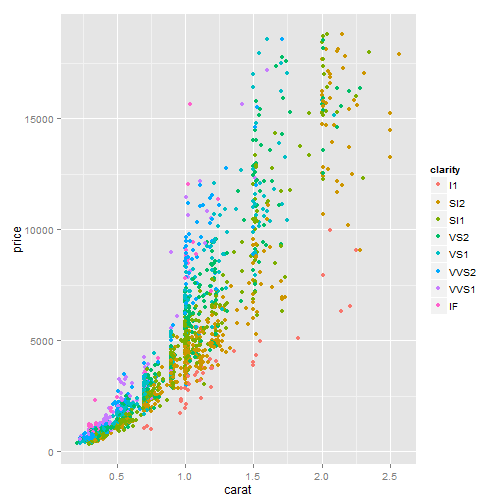## Zev's new workshop slides

You can write the slide in Markdown and HTML. This is bold (markdown) but this is also bold (html).

You might even include inline CSS.

### Quick example with ggplot2

``````> ggplot(diamonds, aes(carat, price))+geom_point(color="firebrick")
``````### Two column example

First plot

``````> ggplot(diamonds, aes(carat, price))+geom_point(color="cadetblue")
``````Second plot

``````> ggplot(diamonds, aes(carat, price, color=clarity))+geom_point()
``````Now I should go back to one column (fullwidth)

• One item
• Two item

This is a paragraph that also gets revealed incrementally.

``````# this is an R code chunk that will be revealed incrementally
x<-1:10
y<-rnorm(10)``````